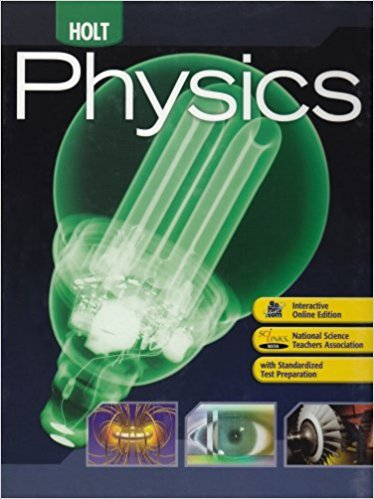×

# Which of the following is the best order-of-magnitudeISBN: 9780030368165 258

## Solution for problem 3 Chapter 1.3

Holt Physics: Student Edition 2009 | 1st Edition

• Textbook Solutions
• 2901 Step-by-step solutions solved by professors and subject experts
• Get 24/7 help from StudySoup virtual teaching assistantsHolt Physics: Student Edition 2009 | 1st Edition

4 5 0 291 Reviews
21
0
Problem 3

Which of the following is the best order-of-magnitude estimate in meters of the height of a mountain? a. 1 m b. 10 m c. 100 m d. 1000 m

Step-by-Step Solution:
Step 1 of 3

r nnntl(S in lprne v\t lSu',rf fIurn & wHCve rnovt v uftlYrrre whdf f wt lrrupre nat{"s pllfion offlt* ) tl ttx obitcl i:il YI h- 6goc,'t*kdv*lY!...

Step 2 of 3

Step 3 of 3

##### ISBN: 9780030368165

Since the solution to 3 from 1.3 chapter was answered, more than 219 students have viewed the full step-by-step answer. Holt Physics: Student Edition 2009 was written by and is associated to the ISBN: 9780030368165. The answer to “Which of the following is the best order-of-magnitude estimate in meters of the height of a mountain? a. 1 m b. 10 m c. 100 m d. 1000 m” is broken down into a number of easy to follow steps, and 29 words. The full step-by-step solution to problem: 3 from chapter: 1.3 was answered by , our top Physics solution expert on 01/19/18, 04:35PM. This textbook survival guide was created for the textbook: Holt Physics: Student Edition 2009, edition: 1. This full solution covers the following key subjects: . This expansive textbook survival guide covers 12 chapters, and 143 solutions.

Unlock Textbook Solution

Which of the following is the best order-of-magnitude

×